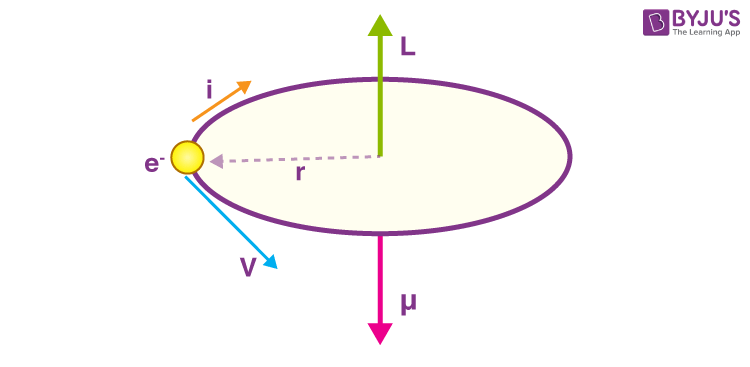# Magnetic Moment of Revolving Electron and Bohr MagnetonLet us consider an electron that is revolving around in a circle of radius r with a velocity v. The charge of the electron is e and its mass is m, both of which are constant. The time period T of the electrons’ orbit is:

$$\begin{array}{l}T = \frac{Circumference}{Velocity} = \frac{2πr}{v}\end{array}$$

The current i due to the motion of the electron is the charge flowing through that time period,

$$\begin{array}{l}i = \frac{-e}{\frac{2πr}{v}}= \frac{-ev}{2πr}\end{array}$$

Note, that the current is in the opposite direction as the electron is negatively charged. The magnetic moment due to a current loop enclosing an area A is given by:

$$\begin{array}{l}μ = iA\end{array}$$

Magnetic moment of an electron:

$$\begin{array}{l}μ= \frac{-ev}{2πr}~ A = \frac{-ev}{2πr}~ π{r^2}\end{array}$$
$$\begin{array}{l}μ = \frac{-erv}{2}\end{array}$$

Let us divide and multiply by the mass of the electron,

$$\begin{array}{l}μ = \frac{-e}{2m_e}~ m_e~ vr\end{array}$$

We know that the angular momentum L is given by:

$$\begin{array}{l}L = mvr\end{array}$$
Thus, we can write,

$$\begin{array}{l}μ = \frac{-e}{2m_e}~ L\end{array}$$

Since, the angular momentum is given by the right-hand rule with respect to the velocity and the current in the opposite direction hence, the negative sign shows that the two quantities are on opposing directions as shown in the figure,

$$\begin{array}{l}\overrightarrow{μ} = \frac{-e}{2m_e}~\overrightarrow{L}\end{array}$$

This is an important result as the magnetic moment is only dependent upon the angular momentum. This is why the orbital angular momentum and orbital magnetic moment terms are used interchangeably. The same is true for the spin angular moment.

For an electron revolving in an atom, the angular momentum is quantized as proposed by Niels Bohr.

The angular momentum is given by:

$$\begin{array}{l}L = n~ \frac{h}{2π},~n = 0,~±1,~±2~…\end{array}$$

Where n is the orbit quantum number and h is the Planck’s constant,

$$\begin{array}{l}μ = n~\frac{-e}{2m_e}~\frac{h}{2π}\end{array}$$
$$\begin{array}{l}μ = -n~\frac{eh}{4πm_e}\end{array}$$

The quantity that multiplies with n is constant and is known as the Bohr Magneton µB.

$$\begin{array}{l}μ_B = \frac{eh}{4πm_e} = 9.27~×~10^{-27}\,J⁄T\end{array}$$

Following is the table of links related to magnetic characteristic:

The Bohr Magneton is used very widely to express magnetic moments at the atomic scale. The expression we obtained is good for only simple atoms like hydrogen and does not predict all the magnetic moment states of an electron. As you would have learned in chemistry, the electron does not really revolve around the nucleus. Instead, the electron’s orbital magnetic moment is obtained by virtue of being trapped in the nuclei (plural of nucleus) potential well.

The spin and orbital magnetic moments of atoms combine vectorially in a sample to produce the net magnetic moment of that particular sample. It is these magnetic moments obtained by the combination of orbital and spin magnetic moments that determine the magnetic properties of the materials.